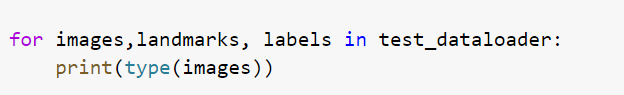# Tutorial WRITING CUSTOM DATASETS, DATALOADERS AND TRANSFORMS tutorial

Good afternoon!
I have questions about the following tutorial:

I have a similar dataset (images + landmarks). I’ve built the custom dataloader following the tutorial and checked the types of dataloader components (torch.float64 for both images and landmarks).

Then I applied the dataloader to the classification model with this training class:

``````class Trainer():

def __init__(self,criterion = None,optimizer = None,schedular = None):

self.criterion = criterion
self.optimizer = optimizer
self.schedular = schedular

train_loss = 0.0
train_acc = 0.0
images = images.to(device)
landmarks = landmarks.to(device)
labels = labels.to(device)
``````

I won’t be elaborating further because the training crushes at images = images.to(device) with the following error: AttributeError: ‘str’ object has no attribute 'to’

I don’t understand where this string is coming from if all the dataloader components are torch.float64.
I went back to check the initial data: in the tutorial, the landmarks are summarized in a pandas dataframe with landmark values as int64 and image name as “object”.
In my summary dataframe image name is an “object” as well and landmarks are numpy.float64. Again, no strings anywhere…

I will share the code for creating a summary datatable in the first comment.
Appreciate any ideas!

``````def load_landmarks(path, header):
filename = path.split("\\")[-1].replace("txt", "png")
instance = np.swapaxes(pd.read_csv(path, delimiter ="\t", index_col=False).values, 0,1)
data_list = list(zip(instance, instance))
flattened = [item for sublist in data_list for item in sublist]
flattened.insert(0, filename)
frame = pd.DataFrame(np.array(flattened).reshape(-1,len(flattened)), columns = header)
return frame
``````
``````def data_summary(path, num_landmarks):
for i in range(num_landmarks):
labels_lst = []
for label in os.listdir(path):
for roots, dirs, filenames in os.walk(os.path.join(path, label)):
for file in filenames:
filename = os.path.splitext(file)
if filename == ".png":
label = label
cat_number = file.split("_")
labels_lst.append(tuple((int(label), cat_number)))
shutil.copy(os.path.join(roots, file), images_path)
if filename == ".txt":
temp_path = os.path.abspath(os.path.join(path, label, file))
data_table = data_table.append(landmark)
labels = [i for i in labels_lst]
cat_numbers = [i for i in labels_lst]

data_table.insert(0, 'cat_number', cat_numbers)
data_table.insert(1, 'label', labels)
data_table['cat_number'] = data_table['cat_number'].astype('int64')
return data_table
``````
``````class FaceLandmarksDataset(Dataset):
def __init__(self, data_frame, root_dir, transform=None):
self.data_frame = data_frame
self.root_dir = root_dir
self.transform = transform

def __len__(self):
return len(self.data_frame)

def __getitem__(self, idx):
if torch.is_tensor(idx):
idx = idx.tolist()

img_name = os.path.join(self.root_dir, self.data_frame.iloc[idx, 2])
landmarks = self.data_frame.iloc[idx, 3:]
landmarks = np.array([landmarks])
landmarks = landmarks.astype('float').reshape(-1, 2)
labels = self.data_frame.iloc[idx, 1].reshape(1)
sample = {'image': image, 'landmarks': landmarks, 'labels': labels}

if self.transform:
sample = self.transform(sample)

return sample
``````

Print the `dtypes` of the `sample` in the `__getitem__` before and after applying `self.transform` on them and check if some of them are strings.

I’ve checked that - maybe I did something wrong?

Before transformation:

``````face_dataset = FaceLandmarksDataset(full_data_table, images_path)
for i in range(len(face_dataset)):
sample = face_dataset[i]
print(i, sample['image'].shape, sample['landmarks'].shape, sample['labels'].shape)
print(i, sample['image'].dtype, sample['landmarks'].dtype, sample['labels'].dtype)

# image - (1000, 1000, 4), uint8
# landmarks  - (48, 2), float64
# label -  (1,), int64
``````

After transformation:

``````scale = Rescale(224)
transformed_dataset = FaceLandmarksDataset(full_data_table, images_path,
transform=transforms.Compose([scale, ToTensor()]))

for i in range(len(transformed_dataset)):
sample = transformed_dataset[i]

print(i, sample['image'].size(), sample['landmarks'].size(), sample['labels'].size())
print(i, sample['image'].dtype, sample['landmarks'].dtype, sample['labels'].dtype)

# image - [4, 224, 224]), torch.float64
# landmarks  - torch.Size([48, 2]), torch.float64
# label -  torch.Size(), torch.int64
``````

The `dtypes` look correct. Are you seeing the error in a specific iteration or directly in the first one?
In the former case, you could keep the print statements, start the training, and check if the `dtype` changes in one iteration. I would recommend not to shuffle the dataset so that you could check which index was used to create the data.

The error shows up directly in the first iteration and I don’t shuffle the data at Dataloader stage. the dataset, fed into dataloader already comes shuffled.

OK, that’s interesting as it seems that iterating `transformed_dataset` creates the expected tensors while iterating the `DataLoader` returns a string and causes the error.
Could you use a batch size of 1 and iterate the `DataLoader` again with the same print statements?

Is it possible that somewhere during data processing dataloader gets an image name instead of an actual image? Where can it be (maybe data_summary function?)

If the strings are not found anymore, `images = images.to(device)` wouldn’t be failing with `AttributeError: ‘str’ object has no attribute 'to’`, would it?

In case it’s still failing you, it seems you are hitting these issues now:

• the data has definitely the correct `dtype` in the `__getitem__` before the `return` statement
• inside the `DataLoader` loop the `images` are strings and the `to` call is failing

If so, then the place to look next into would be the `collate_fn` and I assume you might be using a custom one. By default this method stacks the samples returned by `Dataset.__getitem__` into a batch and does not manipulate the data.
However, I don’t know if a custom `collate_fn` is used which might not be interacting nicely with the returned `dict`.

Another idea: are you indexing the `dict` with the right keys or are you iterating the returned `dict` and are trying to use the `keys` as the data?
Remove the `dict` usage for the sake of debugging and just return the samples:

``````        sample = {'image': image, 'landmarks': landmarks, 'labels': labels}

if self.transform:
sample = self.transform(sample)

return sample['image'], sample['landmarks'], sample['labels']
``````

Thanks for your response. I tried the dict manipulation you suggested, dtypes are still torch floats.

I’ve just found the string. The for-loop in Trainer class “for images,landmarks, labels in train_dataloader: …” is iterating incorrectly over the dataloder.Now I need to figure out the way to fix it